Question

# Find the zeros for the given polynomial function and give the multiplicity for each zero. State...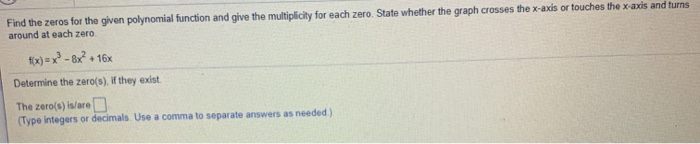Find the zeros for the given polynomial function and give the multiplicity for each zero. State whether the graph crosses the x-axis or touches the x-axis and turns around at each zero fix)=x2-8x? + + 16x Determine the zero(s). If they exist. The zero(s) is/are D (Type integers or decimals. Use a comma to separate answers as needed)
Find the zeros for the given polynomial function and give the multiplicity for each zero. State whether the graph crosses the x-axis or touches the x-axis and turns around at each zero fix)=x2-8x? + + 16x Determine the zero(s). If they exist. The zero(s) is/are D (Type integers or decimals. Use a comma to separate answers as needed)

f(x) = x^3 - 8x^2 + 16x

Setting f(x) = 0

x^3 - 8x^2 + 16x = 0

x ( x^2 - 8x + 16 ) = 0

x ( x - 4)^2 = 0

Setting each factor to 0

x = 0 , x - 4 = 0

x - 4 = 0

x = 4

 The zeros are 0 ( multiplicity 1 ) , 4 ( multiplicity 2 )

The graph crosses the x axis at x = 0

The graph touches the x axis at x = 4

#### Earn Coins

Coins can be redeemed for fabulous gifts.

Similar Homework Help Questions
• ### 2.25 & Skill Builder Question Hels and the zeros for the polynomial function and give the...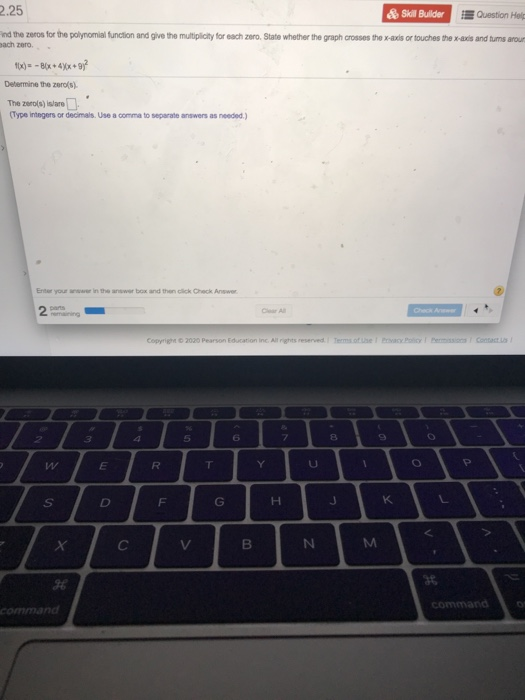2.25 & Skill Builder Question Hels and the zeros for the polynomial function and give the multiplicity for each zoro. State whether the graph crosses the x-axis or touches the x-axis and tums aroun Bach zero 1x) = - B(x + 4yx +91 Determine the zero(s) The zero(s) islano . (Type Integers or decimals. Use a comma to separate answers as needed.) Enter your in the answer box and then click Check Answer 2 CA Copyright 2020 Pearson Education Inc....

• ### Analyze the polynomial function f(x)=x2 + x2 - 20x. Complete parts (a) through (c). (a) Find...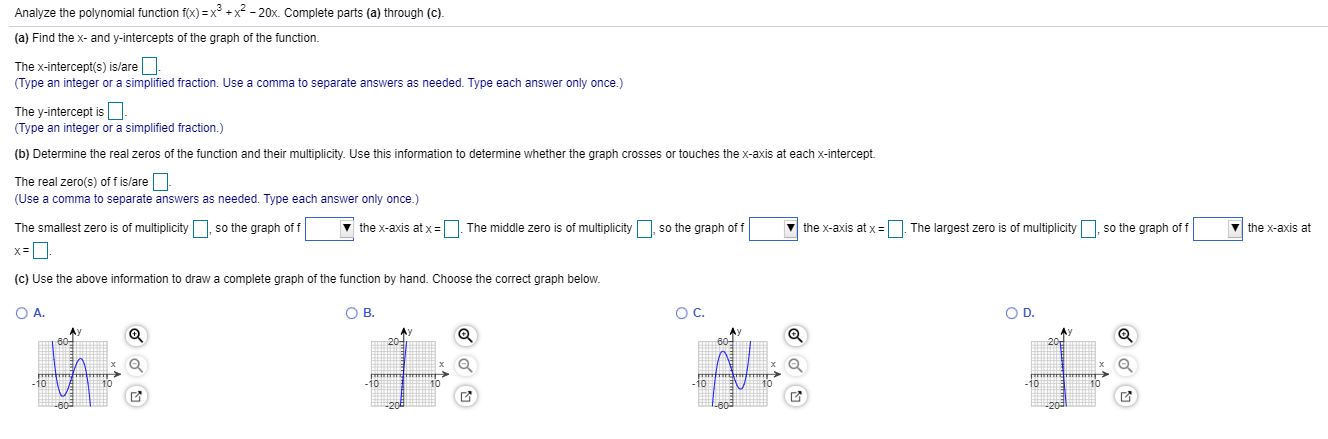Analyze the polynomial function f(x)=x2 + x2 - 20x. Complete parts (a) through (c). (a) Find the x- and y-intercepts of the graph of the function. The x-intercept(s) is/are - (Type an integer or a simplified fraction. Use a comma to separate answers as needed. Type each answer only once.) The y-intercept is - (Type an integer or a simplified fraction.) (b) Determine the real zeros of the function and their multiplicity. Use this information to determine whether the graph...

• ### For the polynomial, list each real zero and its multiplicity

For the polynomial, list each real zero and its multiplicity. Determine whether the graph crosses or touches the x-axis at each x -intercept. f(x) = 3(x + 6)(x + 5)4

• ### for the polynomial, list each real zero and its multiplicity

for the polynomial, list each real zero and its multiplicity. determine whether the graph crosses or touches the x-axis at each x-intercept. f(x)=1/2x^4(x^2-5)(x+1)

• ### Find the zeros of the polynomial function and state the multiplicity of each zero. (Enter your...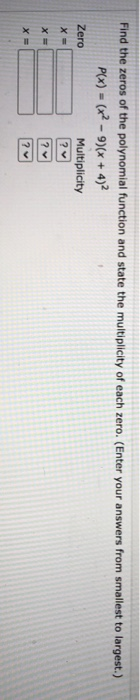Find the zeros of the polynomial function and state the multiplicity of each zero. (Enter your answers from smallest to largest.) P(x) = (x2 - 9)(x + 4)2 Zero Multiplicity ? X = X = ? х ? Evaluate the determinant by expanding by cofactors. 3-4 6 3-4 0 0 5 MOO

• ### Find the zeros of the polynomial function and state the multiplicity of each. f(x) = 4x²-x²...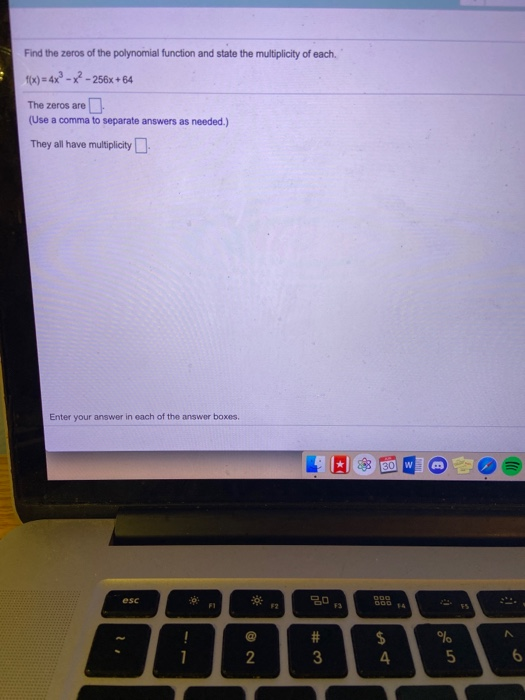Find the zeros of the polynomial function and state the multiplicity of each. f(x) = 4x²-x² - 256x+64 The zeros are (Use a comma to separate answers as needed.) They all have multiplicity Enter your answer in each of the answer boxes 30 W esc 30 ... 52 73 DOD # 3 \$ 4 % 5 6 N

• ### 2.3 2.5

Find the zeros for the polynomial function and give the multiplicity for each zero. State whether the graph crosses the x-axis or touches the x-axis and turns around at each zero.f(x)= -2(x-9)(x+3)2

• ### Find the x-intercepts of the polynomial function State whether the graph crosses the x-axis, or touches...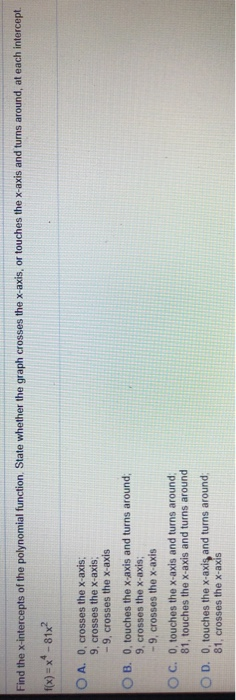Find the x-intercepts of the polynomial function State whether the graph crosses the x-axis, or touches the x-axis and turns around, at each intercept. f(x) = ** -81x? O A. O, crosses the x-axis: 9. crosses the x-axis: -9, crosses the x-axis OB. O, touches the x-axis and turns around; 9, crosses the x-axis; -9, crosses the x-axis OC. 0, touches the x-axis and turns around 81, touches the x-axis and turns around OD. O, touches the x-axis and turns...

• ### A polynomial function f(x) has a zero of 3 with multiplicity 2

A polynomial function f(x) has a zero of 3 with multiplicity 2. (1)since the zero is 3, the graph crosses the y-axis at 3? (2) since the zero is 3, the graph goes up to the right? (3) since the multiplicity is 2, the graph crosses the x-axis? (4) since the multiplicity is 2, the graph touches but does not cross the x-axis? Please help me with this!!!

• ### Analyze the polynomial function fix) = x(x 3)(x + 7) using parts (a) through (e). la...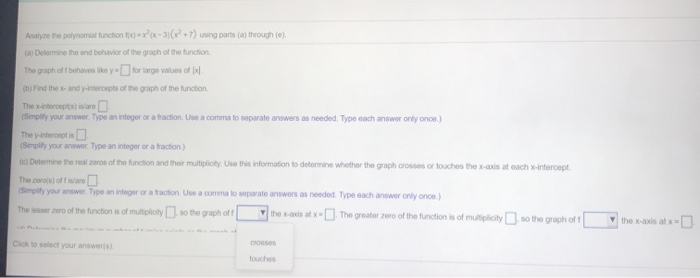Analyze the polynomial function fix) = x(x 3)(x + 7) using parts (a) through (e). la Determine the end behavior of the graph of the function The graph off behaves like y- for large values of Ix/ (b) Find the x-and intercepts of the graph of the function Thexintercepts) isare (Simply your answer. Type an integer or a tractionUse a comma to separate answers as needed. Type oath answer only once.) The interceptis (Simplify your answer. Type an integer or...